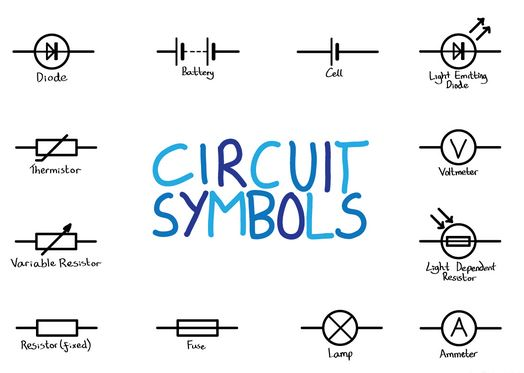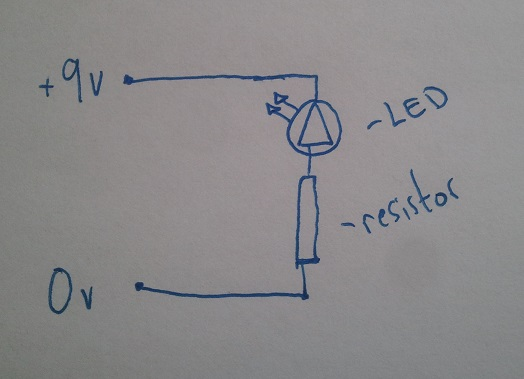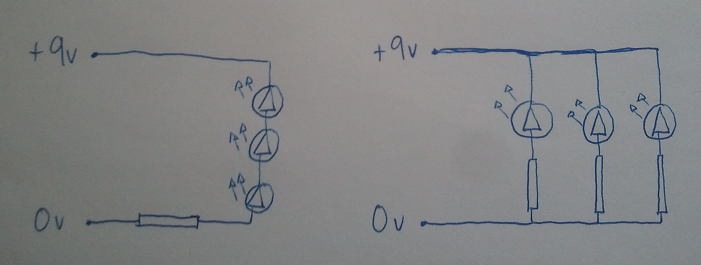# Circuit Diagrams

In this lesson we are going to take a loot at how to draw out circuit diagrams on paper, and then how to convert those to actual built circuits on the breadboad.Remember the symbols for some of the circuit components? You can see them here at this link.

Here is a circuit diagram for a simple circuit with one LED.Here are two circuit diagrams, can you figure out which one is a parallel circuit and which one is a series circuit?Now how about these circuit diagrams?
Can you identify all the components on the circuit?
If not then look back in your notes.